# Short Questions With Answers - Market Equilibrium Notes | Study Economics Class 11 - Commerce

## Commerce: Short Questions With Answers - Market Equilibrium Notes | Study Economics Class 11 - Commerce

The document Short Questions With Answers - Market Equilibrium Notes | Study Economics Class 11 - Commerce is a part of the Commerce Course Economics Class 11.
All you need of Commerce at this link: Commerce

Q.1. Define equilibrium price.
Ans.
Equilibrium price is the price at which the quantity demanded is equal to the quantity supplied. It is obtained at the point of intersection of demand and supply curves.

Q.2. Define equilibrium.
Ans.
Equilibrium refers to the state of rest. It is a position from which there is no net tendency to move.

Q.3. Define market equilibrium.
Ans.
Market is said to be in equilibrium when market supply is equal to the market demand. At equilibrium, there is no tendency for price or quantity to change. There is no excess demand or excess supply at equilibrium.

Q.4. Give the meaning of excess demand for a product.
Ans.
Excess demand refers to a situation when consumers want more than what producers are willing to supply at given prices. Excess demand creates competition among buyers and pushes the price up.

Q.5. Give the meaning of excess supply of a product.
Ans.
Excess supply to a situation when quantity demanded is less than the quantity supplied at given prices. It creates competition among the sellers and causes the price to fall.

Q.6. Explain the process of determination of equilibrium price under perfect competition with the help of suitable table and diagram.
Ans.
Equilibrium price is determined where market demand and market supply are equal. We can show this by the following table.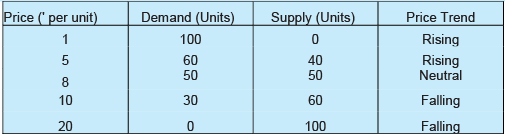In the table, when the price of the good is very less, i.e. ' 1 per unit then its demand is very high (100 units). However, no seller is ready to sell on this price and hence, supply is zero. Excess demand creates competition among the buyers and pushes the price up. When price is ' 5 per unit, the quantity demanded is 60 units while quantity supplied is 40 units. Again, there is excess demand by 20 units. Price would continue to increase. When price is ' 8 per unit, the quantity demanded is equal to the quantity supplied at 50 units. This price is called Equilibrium Price.
Similarly, when the price of the good is ' 20 per unit then its supply is very high (100 units). However, no buyer is ready to buy on this price and hence, demand is zero. Excess supply creates competition among the sellers and pulls the price down. When price is ' 10 per unit, the quantity supplied is 60 units while quantity demanded is 30 units. Again, there is excess supply by 30 units. Price would continue to fall.

Q.7. If at a given price of a commodity, there is excess demand, how will the equilibrium price be reached? Explain with the help of a diagram.
Ans.
When there is an excess demand for a good then the given price of a good is lower than the equilibrium price. The equilibnum pnce can be attained by reaching the point of interaction between market demand and supply curves. This can be explained with the help of the given diagram.
In this diagram, quantity is shown on X axis and price is shown on Y axis. SS is the supply curve and DD is the demand curve. At the initial price of OP!, the quantity demanded is more than the quantity supplied (OQ2 > OQ). There is an excess demand equal to QQ or AB.
Excess demand creates competition among the buyers and pushes price up. Rise in price decreases the quantity demanded (from B to E) and increases the quantity supplied (from A to E ).These changes continue till price rises to OP . The equilibrium is attained at the point E , where the quantity demanded and quantity supplied are equal. OP is the equilibrium price and OQ is the equilibrium quantity.

Q.8. How does an increase in the price of a substitute good in consumption affect the equilibrium price?
Ans.
An increase in the price of a substitute good in consumption leads to an increase in the equilibrium price.

Q.9. How does an increase in input price affect the equilibrium quantity exchanged in the product market?
Ans.
An increase in input price leads to a decrease in the equilibrium quantity exchanged in the product market.

Q.10. How does a favourable change in taste affect the market price and the quantity exchanged?
Ans.
A favourable change in taste leads to an increase in the market price and the quantity exchanged.

Q.11. How does a cost-saving technological progress affect the market price and the quantity exchanged?
Ans.
A cost saving technological progress leads to a fall in the market price and increase in the quantity exchanged.

Q.12. How does an increase in excise tax rate affect the market price and the quantity exchanged?
Ans.
An increase in excise tax rate leads to an increase in the market price and decrease in the quantity exchanged.

Q.13. When will an increase in demand imply an increase in price but no change in quantity supplied?
Ans.
An increase in demand will imply an increase in price, but no change in quantity supplied when the supply curve is perfectly inelastic.

Q.14. What is the impact of imposing price ceiling below the equilibrium price?
Ans. Imposition of price ceiling below the equilibrium price leads to an excess demand.

Q.15. What is the impact of imposing price floor above the equilibrium price?
Ans. Imposition of price floor above the equilibrium price leads to an excess supply.

Q.16. Give a few examples of items on which price ceiling is imposed?
Ans. Price ceiling is generally imposed on necessary items such as wheat, rice, kerosene and sugar.

Q.17. Explain the effects of an increase in demand and supply of a commodity on its price.
Ans.
Effects of Changes in Demand The effect on price when there is an increase or decrease in demand and the supply remains unchanged can be explained with the help of the diagram.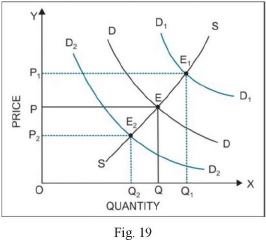DD is the demand curve and SS is the supply curve, which remains fixed. The initial price is OP. If the demand increases from DD to D D , the price rises to OP!. Similarly, with the decrease in demand from DD to D2D2> the price falls to OP2.
Effects of Changes in Supply The effect on price when there is an increase or decrease in supply and the demand remains unchanged can be explained with the help of the diagram.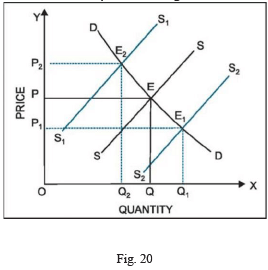SS is the supply curve and DD is the demand curve, which remains fixed. The initial price is OP. If the supply increases from SS to S(S , the price falls to OP . Similarly, with the decrease in supply from S2S2 to SS, the price rises to OP2.

Q.18. Explain with the help of a diagram the effect of a leftward shift of supply curve of a commodity on its equilibrium price and quantity.
Or
Market for a good is in equilibrium. Suppose supply decreases. Giving reasons, explain its effect on equilibrium, price and quantity. Use diagram.
Ans.
A change in the supply curve affects the equilibrium price and quantity. A decrease in the supply or a leftward shift in the supply curve causes an increase in the equilibrium price and a decrease in the equilibrium quantity.
In the diagram, when the supply curve shifts leftwards from SS to S^, equilibrium quantity decreases from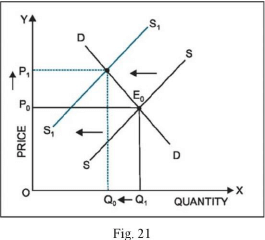OQ0 to OQ and equilibrium price increases from OP0 to OP,. The new equilibrium point will be at E .

Q.19. How is the equilibrium price of a commodity affected by a leftward shift of the demand curve? Explain with the help of a diagram.
Ans.
The equilibrium price of a good falls due to a leftward shift in the demand curve. It can be explained with the help of the diagram.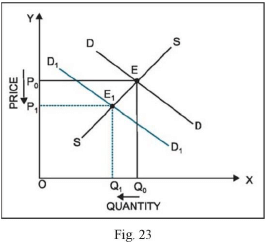In this diagram, quantity is shown on X axis and price is shown on Y axis. SS is the supply curve and DD is the demand curve. At the point of intersection of DD and SS curves, the equilibrium price and equilibrium quantity are OP and OQ respectively. When the demand curve shifts from DD to D D , the equilibrium price falls from OP to OP . The equilibrium quantity also falls from OQ to OQ!. Thus, a leftward shift in the demand curve leads to a fall in the equilibrium price.

Q.20. Market for a good is in equilibrium.What is the effect on equilibrium price and quantity if both market demand and market supply of the good increase in the same proportion? Use diagram.
Ans.
Equilibrium price and quantity are affected by simultaneous shifts in the demand and supply curves. The impact on equilibrium quantity can be predicted but the equilibrium price may change, if at all, in either direction depending on the slopes of both the curves and the magnitude of shift.
If both demand and supply of a good increase in the same proportion, there will be no change in the equilibrium price.
However, the equilibrium quantity will increase. This can be explained with the help of a diagram.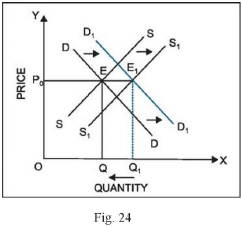In the diagram, quantity is shown on X axis and price is shown on Y axis. SS is the initial supply curve and DD is the initial demand curve. At the point of intersection of DD and SS curves, the equilibrium price and equilibrium quantity are OPQ and OQ respectively. When the demand curve shifts from DD to DD and the supply curve shifts from SS to SS , equilibrium quantity increases from OQ to OQ , but equilibrium price remains unchanged at OP0.

Q.21. Explain the effects of ‘increase’ in supply of a good on its equilibrium and equilibrium quantity. Use diagram.
Ans.
Increase in supply implies that more quantity is being supplied at the given price. The supply curve will shift to the right from SS to SS . This creates excess supply (EA) at price OP . Since the firms are not able to sell what they produce, competition among the firms leads to a fall in price. Fall in price increases the quantity demanded (from E to E ) and decreases the quantity supplied (from A to E ). These changes continue till price falls to OP . OP is the new equilibrium price and OQ is the new equilibrium quantity.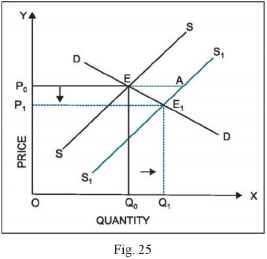Q.22. Explain the effects of ‘increase’ in supply of a good on its equilibrium price and equilibrium quantity with the help of a schedule.
Ans.
Market for a good is in equilibrium only when the demand for the good is equal to the supply of the good. The main causes of an increase in supply curve include fall in input prices, technological advancement, government policy (subsidies), etc. The chain of effects of an increase in supply can be explained with the help of the following numerical example: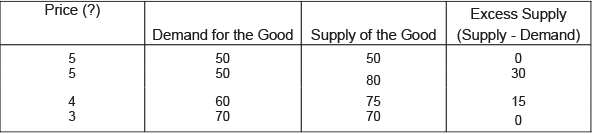In the table above, the market is in equilibrium at price of ' 5. At this price, quantity demanded is equal to the quantity supplied at 50 units. Suppose at the price of ' 5, supply increases from 50 to 80 units (say, due to fall in input prices), while demand remains the same at 50 units. This gives rise to an excess supply of 30 units (80 - 50). Excess supply creates competition among the sellers and pulls the price down. The price, therefore, decreases from ' 5 to ' 4. A fall in price induces the buyers to buy more, and thus, increases the quantity demanded from 50 to 60 units. Also, a fall in price induces the sellers to sell less, and thus, decreases the quantity supplied from 80 to 75 units. These changes continue till price falls to ' 3, where quantity demanded is again equal to the quantity supplied at 70 units. Thus, the new equilibrium price and quantity are ' 3 and 70 units respectively. Equilibrium price has decreased while equilibrium quantity has increased.

Q.23. How will a fall in the price of tea affect the equilibrium price of coffee? Explain the chain of effects.
Ans.
Substitute goods are those goods which can be used in place of one another. Here, tea and coffee are substitute goods. Thus, a fall in the price of tea will cause the demand for coffee to fall. Thus, the demand curve for coffee will shift leftwards. Accordingly, the equilibrium price and quantity will change. The impact of a fall in the price of tea on the equilibnum price and quantity exchanged of coffee can be explained with the help of a diagram.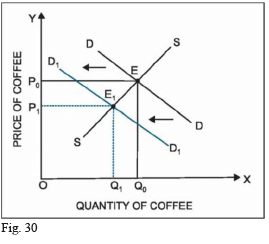In the diagram, the point of initial equilibrium is E where the demand curve DD and supply curve SS intersect each other. At this point, the equilibrium price of a coffee is OPQ and the equilibrium quantity exchanged is OQ0. A decrease in the price of tea causes the demand curve for coffee to shift leftwards from DD to D(Dr Consequently, the equilibrium price and quantity of coffee decrease. The new point of equilibrium is E .
Corresponding to this point, the new equilibrium price and quantity are OP, and OQ respectively.

The document Short Questions With Answers - Market Equilibrium Notes | Study Economics Class 11 - Commerce is a part of the Commerce Course Economics Class 11.
All you need of Commerce at this link: CommerceUse Code STAYHOME200 and get INR 200 additional OFF

### Up next## Economics Class 11

201 videos|195 docs|64 tests

### Up nextTrack your progress, build streaks, highlight & save important lessons and more!

,

,

,

,

,

,

,

,

,

,

,

,

,

,

,

,

,

,

,

,

,

;